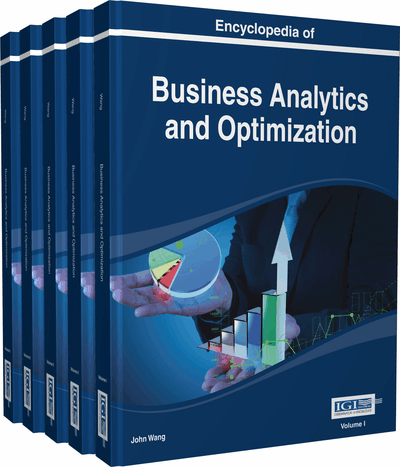# The Practice of Structural Equation Modeling

Ho Yin Wong (Deakin University, Australia), Jason Sit (Bournemouth University, United Kingdom), and Jia-Yi Hung (Tzu Chi College of Technology, Taiwan)
DOI: 10.4018/978-1-4666-5202-6.ch225
Available
\$29.50
No Current Special Offers

## Abstract

The aim of this chapter is to act as a point of reference for researchers, especially new users, who would like to use structural equation modeling (SEM) to perform business analytics. SEM is a multipurpose statistical modeling technique that can be applied in various business disciplines and is a useful tool to conduct business analytics. This chapter explains some common terminologies in the SEM literature and states general steps of performing SEM analysis along with an integration of the wide range of views and empirical findings on the topic. The chapter discusses the assumptions of SEM analysis, followed by a three-stage process of conducting, which includes assumptions, specification, evaluation, and modifications.
Chapter Preview
Top

## Background

SEM is a combination of multivariate statistical analyses such as factor analysis and multiple regression analysis. It differs to, and indeed improves upon, traditional factor analysis and regression analysis. Traditional factor analysis is exploratory in nature, whereas factor analysis in SEM is confirmatory. Hence, they are termed exploratory factor analysis (EFA) and confirmatory factor analysis (CFA), respectively. CFA possesses a major advantage over EFA, in that it is driven by theory. In EFA, all variables of factors are entered into statistical software. Based on a chosen mathematical computation method, the statistical software decides the numbers of factors and which variables belong to which factors. Contrary to EFA, the approach of CFA is that researchers decide which variables are designated to which factors. After mathematical computations, various fit indices confirm if the designated variables fit into the theorized factors. SEM also goes beyond multiple regression analysis, which computes regression equations separately. SEM computes variance-covariance equations simultaneously. Hence, it allows holistic estimations of inter-relationships among the latent variables. Furthermore, in SEM, causal modeling can be done with latent variables, as opposed to the composite variable used in multiple regression analysis, with the consequence of being able to specify an error term for each variable. In summary, SEM can be used to identify and test a theoretical model and to fit the model to the data in a parsimonious manner (Kline, 2005; Schumacker & Lomax, 2010).

## Key Terms in this Chapter

Model Modification: Model modification is the changing of the path and/or the items to improve the fit between data and a theoretical model.

Latent Variables: Latent variables are conceptualized constructs that cannot be directly measured.

Model Specification: Model specification is an exercise for the mapping of latent variables in a theoretical model.

Measurement Model: Measurement model shows the underlying structure of the latent variables in a theoretical model.

Absolute Fit Measures: Absolute fit measures state how well a theoretical model fits the sample data.

Relative Fit Measures: Relative fit measures state indices derived from comparisons between the theoretical model and a baseline model.

Structural Model: Structural model shows the causal and correlational links among latent variables in a theoretical model.

Parsimonious Fit Measures: Parsimonious fit measures present indices that make it possible to examine the fit of competing models on a common basis.

Model Evaluation: Model evaluation is an employment of various fit measures to examine the fit between data and theoretical model.

## Complete Chapter List

Search this Book:
Reset